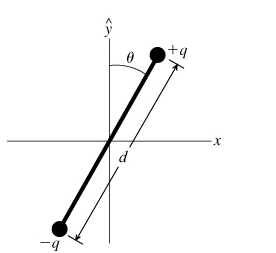# Problem: Consider an electric dipole whose dipole moment (a vector pointing from the negitive charge to the positive charge) is oriented at angle θ with respect to the y axis. There is an external electric field of magnitude E (independent of the field produced by the dipole) pointing in the positive y direction. The positive and negative ends of the dipole have charges +q and –q, respectively, and the two charges are a distance d apart. The dipole has a moment of inertia I about its center of mass. It will help you to imagine that the dipole is free to rotate about a pivot through its center.A) What is the net force Fnet that the dipole experiences due to the electric field?B) What is τcm(θ), the magnitude of the torque that the electric field exerts about the center of mass of the dipole?C) Using the above result, find the potential energy U(θ) associated with the dipole's orientation in the field as a function of the angle theta shown in the figure. Take the zero of the potential to occur when the dipole is at angle π/2; that is, U(π/2) = 0.D) In terms of the dipole moment P, which of the following is an expression for the torque on a dipole in the field E?A. F × EB. E × PC. P · ED. –P · EE) In terms of the dipole moment P, which of the following is an expression for the potential energy of a dipole in the field E?A. P × EB. P ×EC. P · ED. –P · E

###### FREE Expert Solution

The dipole moment is expressed as:

$\overline{){\mathbf{P}}{\mathbf{=}}{\mathbf{q}}{\mathbf{d}}}$

A)

The force acting on the positive charge:

${\mathbit{F}}_{\mathbf{+}\mathbf{q}}\mathbf{=}\mathbit{E}\mathbit{q}$

The force acting on negative charge:

${\mathbit{F}}_{\mathbf{-}\mathbf{q}}\mathbf{=}\mathbf{-}\mathbit{E}\mathbit{q}$

90% (186 ratings)###### Problem Details

Consider an electric dipole whose dipole moment (a vector pointing from the negitive charge to the positive charge) is oriented at angle θ with respect to the y axis. There is an external electric field of magnitude E (independent of the field produced by the dipole) pointing in the positive y direction. The positive and negative ends of the dipole have charges +q and –q, respectively, and the two charges are a distance d apart. The dipole has a moment of inertia I about its center of mass. It will help you to imagine that the dipole is free to rotate about a pivot through its center.A) What is the net force Fnet that the dipole experiences due to the electric field?

B) What is τcm(θ), the magnitude of the torque that the electric field exerts about the center of mass of the dipole?

C) Using the above result, find the potential energy U(θ) associated with the dipole's orientation in the field as a function of the angle theta shown in the figure. Take the zero of the potential to occur when the dipole is at angle π/2; that is, U(π/2) = 0.

D) In terms of the dipole moment P, which of the following is an expression for the torque on a dipole in the field E?

A. F × E
B. E × P
C. P · E
D. –P · E

E) In terms of the dipole moment P, which of the following is an expression for the potential energy of a dipole in the field E?

A. P × E
B. P ×E
C. P · E
D. –P · E